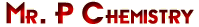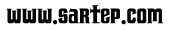Chemical Equations tutorial

A chemical equation is a short hand expression of a chemical reaction. There are two parts to a chemical equation. The reactants are the elements or compounds on the left side of the arrow (-->). The elements and compounds to the right of the arrow are the products.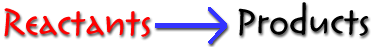Identify the reactants and the products in the equation below.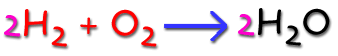Reactants: 2 H2 + O2
Products: 2H2O

Look at the equation above. Notice the two purple 2s. These numbers are called coefficients. Coefficients are used to balance chemical equations.

The blue arrow is a yield sign. It separates the reactants form the products. Most yield signs will be like the one above, but there are some other yield signs you need to know.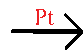A formula written above a yield sign indicates it is a catalyst used to speed up a reaction.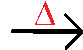A triangle above the yield sign indicates the reaction is heated.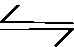Two half arrows pointing in opposite directions indicates that this is a reversible reaction.

The following symbols are written in parenthesis after a formula and represent the state of the element or compound.

 s solid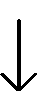solid precipitate is produced (used for products only, not reactants) cr crystalline (used for solid ionic compounds) l liquid aq aqueous (substance is dissolved in water) g gas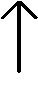gas is produced (used for products only, not reactants)

Identify the following about the equation below: the reactants, the products, the coefficients used, the state of each element or compound.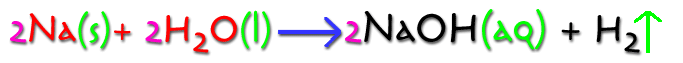Reactants: 2 Na (s) + 2H2O (l)
Products: 2 NaOH (aq) + H2Coefficients: 2,2,2
State:
Na - solid, H2O - liquid, NaOH - aqueous, H2 - gas